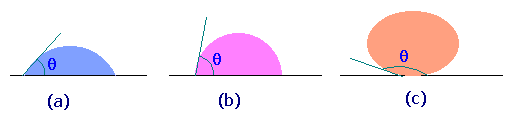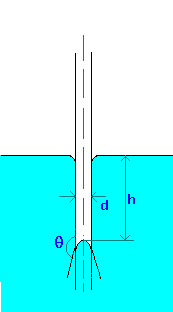### Capillarity

Home -> Lecture Notes -> Fluid Mechanics -> Unit-I

Rise or fall of a liquid in a capillary tube is caused by surface tension and depends on the relative magnitude of cohesion of the liquid and the adhesion of the liquid to the walls of the containing vessel.

Liquids rise in tubes if they wet (adhesion > cohesion) and fall in tubes that do not wet (cohesion > adhesion).

Wetting and contact angle

Fluids wet some solids and do not others.The figure shows some of the possible wetting behaviors of a drop of liquid placed on a horizontal, solid surface (the remainder of the surface is covered with air, so two fluids are present).

Fig.(a) represents the case of a liquid which wets a solid surface well, e.g. water on a very clean copper. The angle q shown is the angle between the edge of the liquid surface and the solid surface, measured inside the liquid. This angle is called the contact angle and is a measure of the quality of wetting. For perfectly wetting, in which the liquid spreads as a thin film over the surface of the solid, q is zero.

Fig.(c) represents the case of no wetting. If there were exactly zero wetting, q would be 180o. However, the gravity force on the drop flattens the drop, so that 180o angle is never observed. This might represent water on teflon or mercury on clean glass.

We normally say that a liquid wets a surface if q is less than 90o and does not wet if q is more than 90o. Values of q less than 20o are considered strong wetting, and values of q greater than 140o are strong nonwetting.

Capillarity is important (in fluid measurments) when using tubes smaller than about 10 mm in diameter.Capillary rise (or depression) in a tube can be calculated by making force balances. The forces acting are force due to surface tension and gravity.
The force due to surface tesnion,
Fs = pdscos(q), where q is the wetting angle or contact angle. If tube (made of glass) is clean q is zero for water and about 140o for Mercury.
This is opposed by the gravity force on the column of fluid, which is equal to the height of the liquid which is above (or below) the free surface and which equals
Fg = (p/4)d2hgr,
where r is the density of liquid.

Equating these forces and solving for Capillary rise (or depression), we find
h = 4scos(q)/(rgd)